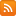CONTÁCTENOS - 91 575 78 24Estás en www.librosingenieriacivilonline.es
Si no encuentra un libro lo buscamos por Ud.
91 575 78 24

## CESTA DE LA COMPRA

### 5 TIENDAS > 1 CESTA

Tiene 0 productos en su cesta Importe total: 0
Classical Beam Theories of Structural Mechanics

# Classical Beam Theories of Structural Mechanics

## Descripción

This book provides a systematic and thorough overview of the classical bending members based on the theory for thin beams (shear-rigid) according to Euler-Bernoulli, and the theories for thick beams (shear-flexible) according to Timoshenko and Levinson.

## Características

• ISBN: 9783030760342
• Páginas: 186
• Tamaño: 17x24
• Edición:
• Idioma: Inglés
• Año: 2021

# Contenido Classical Beam Theories of Structural Mechanics

This book provides a systematic and thorough overview of the classical bending members based on the theory for thin beams (shear-rigid) according to Euler-Bernoulli, and the theories for thick beams (shear-flexible) according to Timoshenko and Levinson. The understanding of basic, i.e., one-dimensional structural members, is essential in applied mechanics. A systematic and thorough introduction to the theoretical concepts for one-dimensional members keeps the requirements on engineering mathematics quite low, and allows for a simpler transfer to higher-order structural members. The new approach in this textbook is that it treats single-plane bending in the x-y plane as well in the x-z plane equivalently and applies them to the case of unsymmetrical bending. The fundamental understanding of these one-dimensional members allows a simpler understanding of thin and thick plate bending members.

Partial differential equations lay the foundation to mathematically describe the mechanical behavior of all classical structural members known in engineering mechanics. Based on the three basic equations of continuum mechanics, i.e., the kinematics relationship, the constitutive law, and the equilibrium equation, these partial differential equations that describe the physical problem can be derived. Nevertheless, the fundamental knowledge from the first years of engineering education, i.e., higher mathematics, physics, materials science, applied mechanics, design, and programming skills, might be required to master this topic.

1 Introduction to Continuum Mechanical Modeling

References

2 Euler–Bernoulli Beam Theory

2.1 Introduction
2.2 Deformation in the x-y Plane
2.2.1 Kinematics
2.2.2 Constitutive Equation
2.2.3 Equilibrium
2.2.4 Differential Equation
2.3 Deformation in the x-z Plane
2.3.1 Kinematics
2.3.2 Constitutive Equation
2.3.3 Equilibrium
2.3.4 Differential Equation
2.4 Comparison of Both Planes
2.5 Unsymmetrical Bending in Both Planes
2.6 Generalized Beam Formulation: Consideration of Tension and Compression
2.7 Shear Stress Distribution Due to Transverse Loads
2.7.1 Solid Sections Beams
2.7.2 Thin-Walled Beams
2.8 Supplementary Problems
References

3 Timoshenko Beam Theory

3.1 Introduction
3.2 Deformation in the x-y Plane
3.2.1 Kinematics
3.2.2 Constitutive Equation
3.2.3 Equilibrium
3.2.4 Differential Equation .
3.3 Deformation in the x-z Plane
3.3.1 Kinematics
3.3.2 Constitutive Equation .
3.3.3 Equilibrium
3.3.4 Differential Equation
3.4 Comparison of Both Planes
3.5 Unsymmetrical Bending in Both Planes
3.6 Supplementary Problems
References

4 Higher-Order Beam Theories

4.1 Introduction
4.2 Deformation in the x-y Plane .
4.2.1 Kinematics .
4.2.2 Constitutive Equation
4.2.3 Equilibrium .
4.2.4 Differential Equation
4.3 Deformation in the x-z Plane
4.3.1 Kinematics
4.3.2 Constitutive Equation
4.3.3 Equilibrium
4.3.4 Differential Equation
4.4 Comparison of Both Planes
4.5 Unsymmetrical Bending in Both Planes
4.6 Supplementary Problems
References

5 Comparison of the Approaches

5.1 Introduction
5.2 Supplementary Problems
References

6 Outlook: Finite Element Approach
References

7.1 Problems from Chap. 2
7.2 Problems from Chap. 3
7.3 Problems from Chap. 4
7.4 Problems from Chap. 5
References

Appendix A: Mathematics
Appendix B: Mechanics
Index

## ACERCA DE LA EMPRESA